Question

# Is the set of polynomials of the form p(x) = a + bra a subspace of...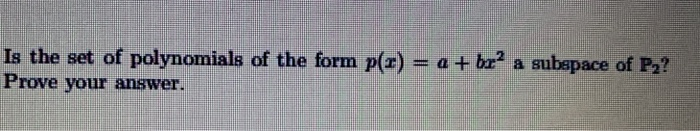Is the set of polynomials of the form p(x) = a + bra a subspace of P2? Prove your answer.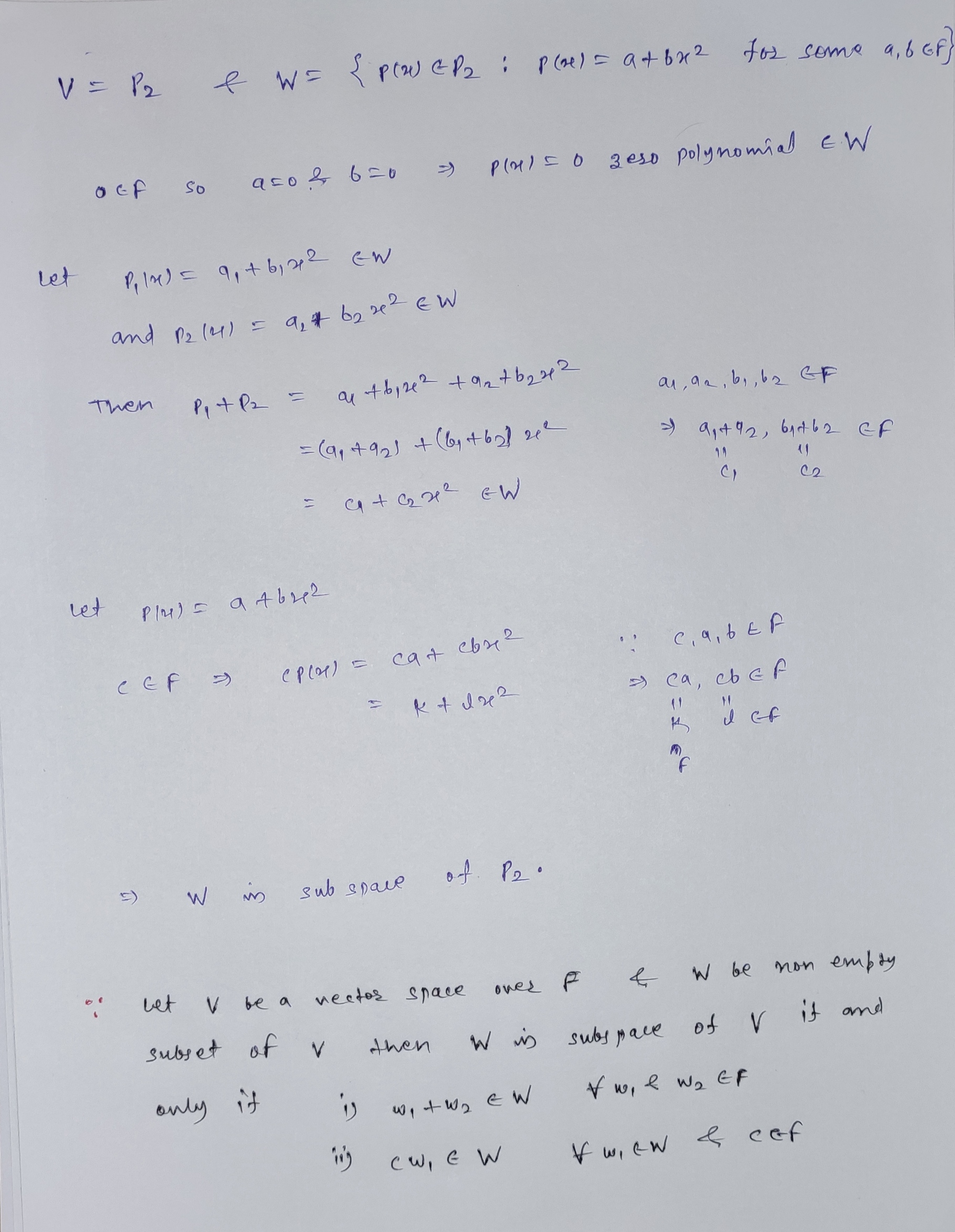#### Earn Coins

Coins can be redeemed for fabulous gifts.

Similar Homework Help Questions
• ### The set of polynomials p(x) = ax2 + bx + c that satisfy p(3) = 0...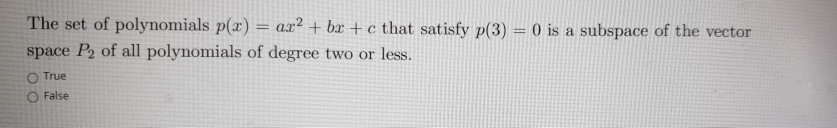The set of polynomials p(x) = ax2 + bx + c that satisfy p(3) = 0 is a subspace of the vector space P2 of all polynomials of degree two or less. O True False

• ### 2. (4) Determine if each of the following is a subspace of P2[x] (the set of...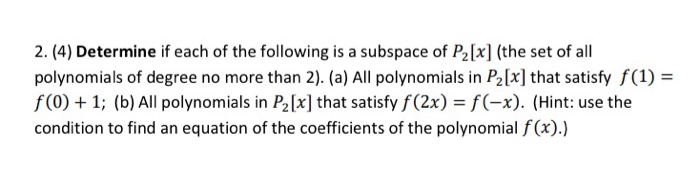2. (4) Determine if each of the following is a subspace of P2[x] (the set of all polynomials of degree no more than 2). (a) All polynomials in P2[x] that satisfy f(1) = f(0) + 1; (b) All polynomials in P2 [x] that satisfy f(2x) = f(-x). (Hint: use the condition to find an equation of the coefficients of the polynomial f(x).)

• ### 6. Let Pm (F) be the vector space of polynomials p(x) = ao + a1x +...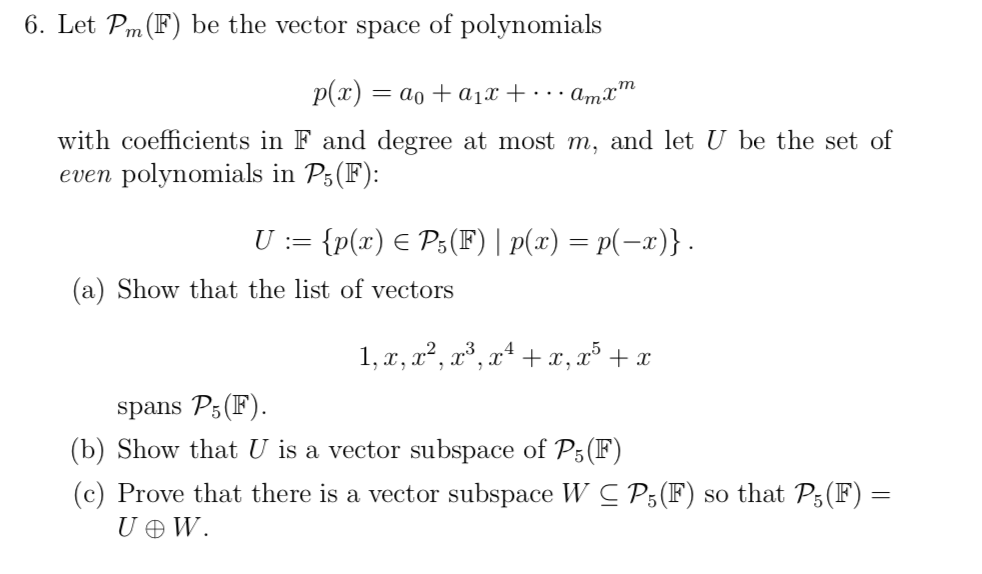6. Let Pm (F) be the vector space of polynomials p(x) = ao + a1x + ... Amx" with coefficients in F and degree at most m, and let U be the set of even polynomials in P5(F): U := {p(x) € P5(F) | P(x) = p(-x)}. (a) Show that the list of vectors 1, x, x², x3, x4 + x, x + x spans P5(F). (b) Show that U is a vector subspace of P5(F) (c) Prove that there...

• ### Let    be the set of third degree polynomials Is    a subspace of   ? Why or why not?...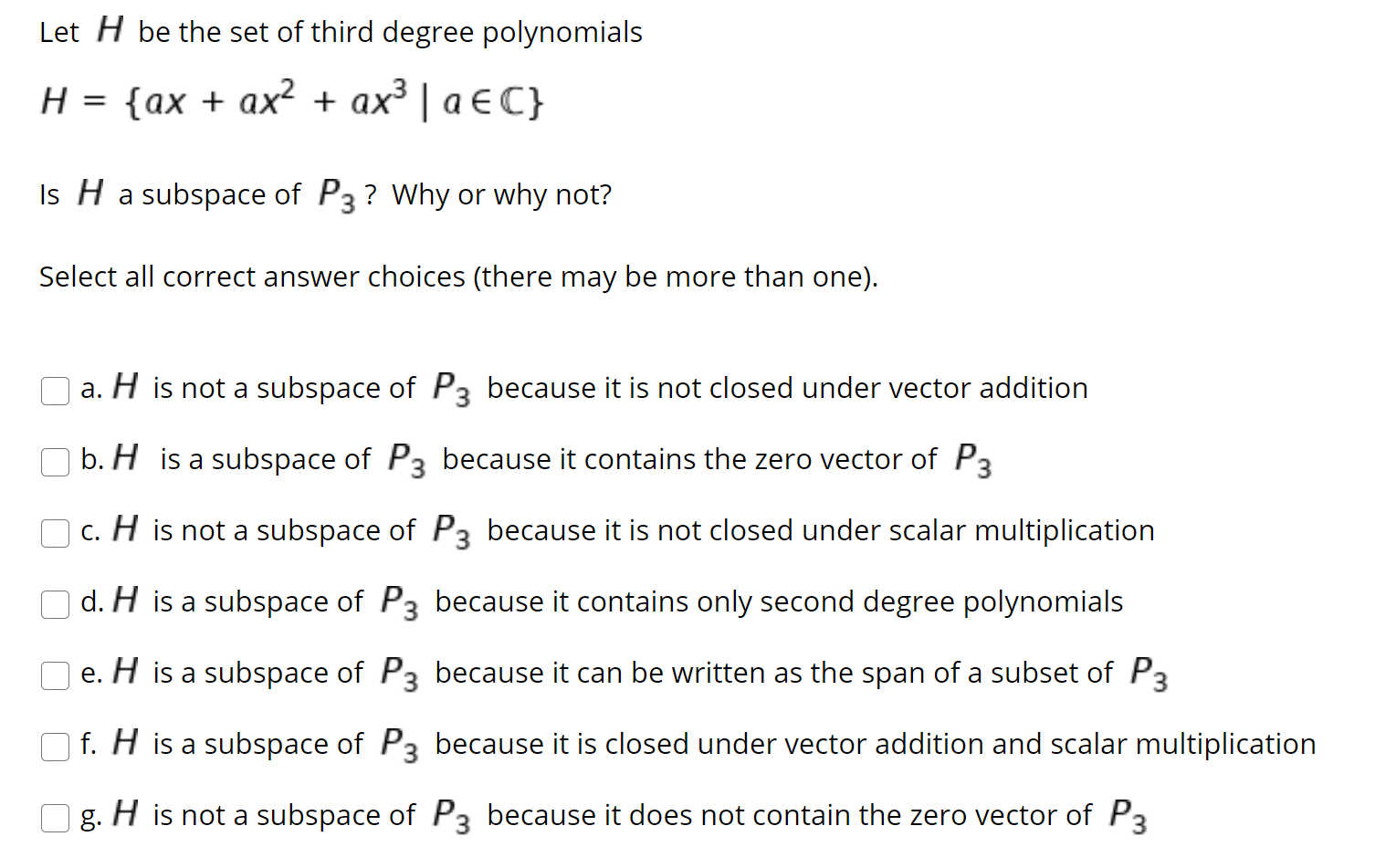Let    be the set of third degree polynomials Is    a subspace of   ? Why or why not? Select all correct answer choices (there may be more than one). a. is not a subspace of    because it is not closed under vector addition b. is a subspace of    because it contains the zero vector of   c. is not a subspace of    because it is not closed under scalar multiplication d. is a subspace of    because it contains only second degree polynomials e. is...

• ### 3. Determine if each set is a subspace of the space of degree < 2 polynomials....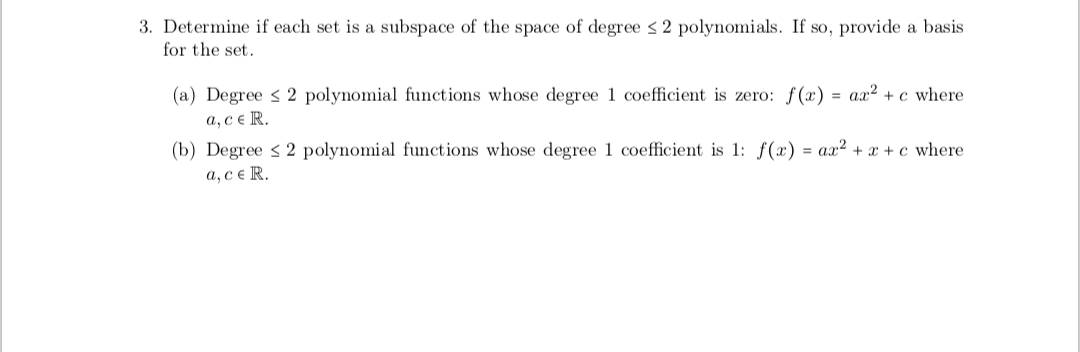3. Determine if each set is a subspace of the space of degree < 2 polynomials. If so, provide a basis for the set. (a) Degree s 2 polynomial functions whose degree 1 coefficient is zero: \$(x) = ax2 + c where a,CER. (b) Degree s 2 polynomial functions whose degree 1 coefficient is 1: f(x) = ax2 + x + c where a,CER.

• ### (a) State whether the following statement is true or false. The follow set is a subspace...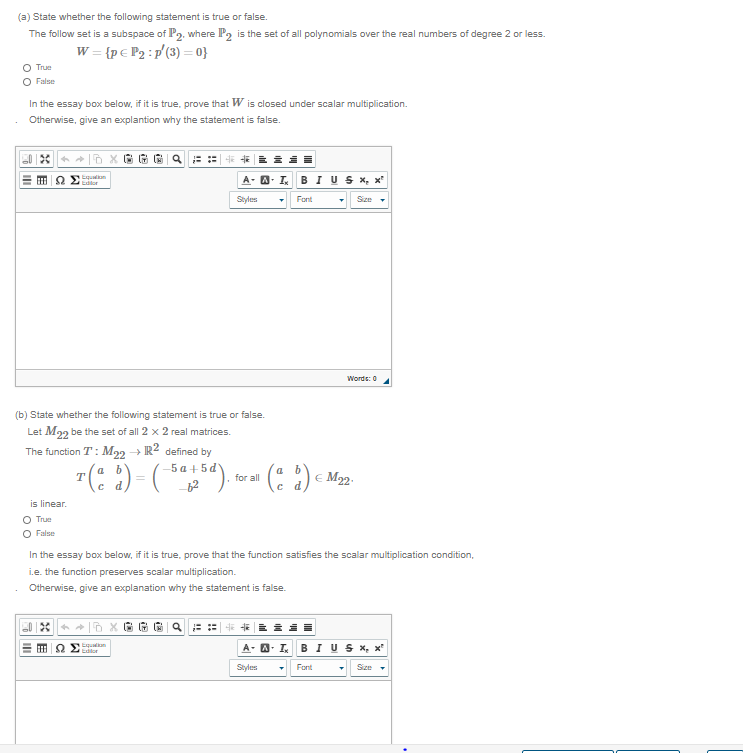(a) State whether the following statement is true or false. The follow set is a subspace of P2, where P2 is the set of all polynomials over the real numbers of degree 2 or less. W={p € P2 :p (3)=0} O True O Fale In the essay box below, if it is true, prove that W is closed under scalar multiplication. Otherwise, give an explantion why the statement is false. XDX HE Editor A-AIBIU S *** Styles Font Size Words:...

• ### Determine if the given set is a subspace of P4. Justify your answer. All polynomials of...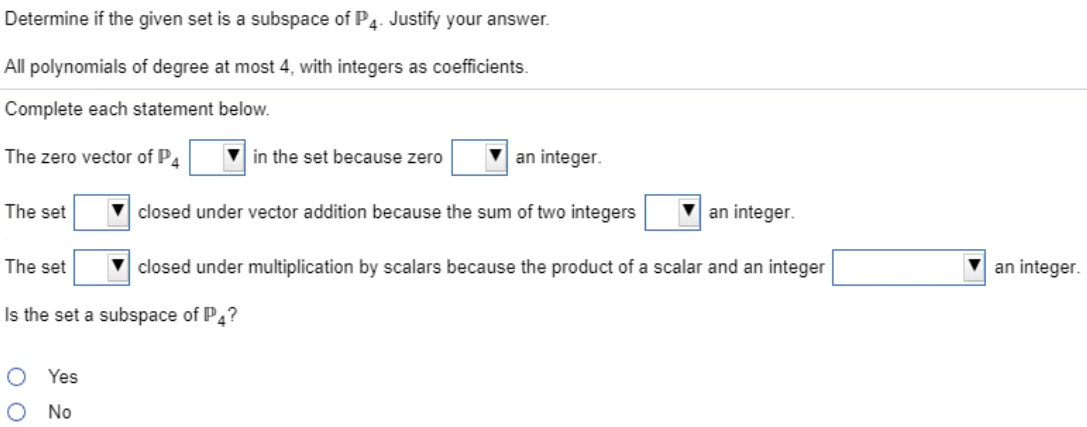Determine if the given set is a subspace of P4. Justify your answer. All polynomials of degree at most 4, with integers as coefficients. Complete each statement below. The zero vector of P4 in the set because zero an integer The set v closed under vector addition because the sum of two integers an integer The set closed under multiplication by scalars because the product of a scalar and an integer an integer Is the set a subspace of P4?...

• ### (1 point) Let P, be the vector space of all polynomials of degree 2 or less,...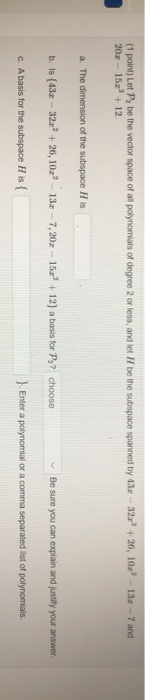(1 point) Let P, be the vector space of all polynomials of degree 2 or less, and let 7 be the subspace spanned by 43x - 32x' +26, 102° - 13x -- 7 and 20.x - 15c" +12 a. The dimension of the subspace His b. Is {43. - 32" +26, 10x - 13.-7,20z - 150 +12) a basis for P2? choose ✓ Be sure you can explain and justify your answer. c. Abasis for the subspace His { }....

• ### (6 pts) 3. Prove whether or not the set of ordered pairs of the form (w...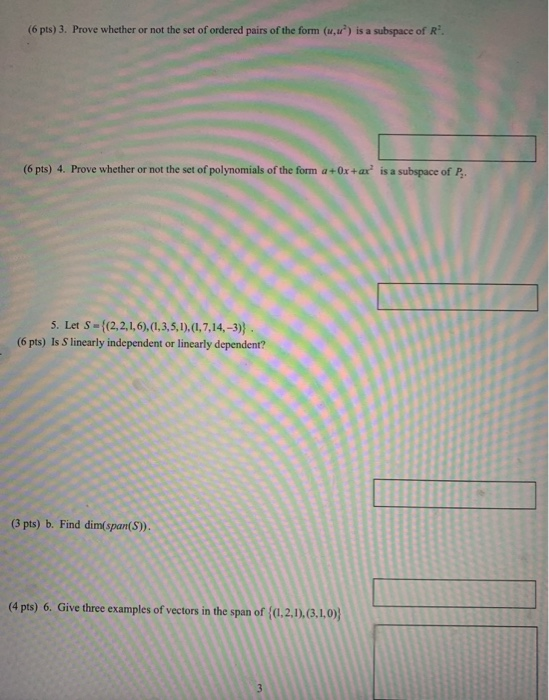(6 pts) 3. Prove whether or not the set of ordered pairs of the form (w ) is a subspace of R'. (6 pts) 4. Prove whether or not the set of polynomials of the form a+Ox+ax is a subspace of P. 5. Let S = {(2,2,1,6),(1,3,5,1),(1,7,14,-3)} . (6 pts) Is S linearly independent or linearly dependent? (3 pts) b. Find (4 pts) 6. Give three examples of vectors in the span of {0.2.1),(3.1.0);

• ### 4. Consider the set of all polynomials p(x, y) in two variables (x,y) € (0,1) (0,...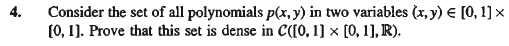4. Consider the set of all polynomials p(x, y) in two variables (x,y) € (0,1) (0, 1). Prove that this set is dense in C([0, 1] x [0, 1], R).